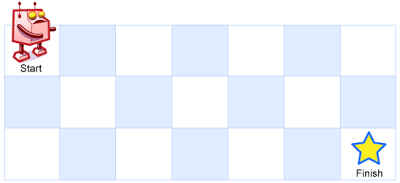# 62 Unique Paths – Medium

### Problem:

A robot is located at the top-left corner of a m x n grid (marked ‘Start’ in the diagram below).

The robot can only move either down or right at any point in time. The robot is trying to reach the bottom-right corner of the grid (marked ‘Finish’ in the diagram below).How many possible unique paths are there?

Above is a 3 x 7 grid. How many possible unique paths are there?

Note: m and n will be at most 100.

### Thoughts:

This is a very basic and easy dynamic programming problem.

Each time the robot needs to make a choice is either go down or go right. Do the optimized solution is the better one from these two choices.

d[i][j] is the number of unique paths to reach point(i,j)

d = 1

d[i][j] = d[i-1][j] +d[i][j-1]

### Solutions:

``````public class Solution {
public int uniquePaths(int m, int n) {
int[][] paths = new int[m][n];
for (int i = 0; i < m; i ++){
paths[i] = 1;
}
for (int j = 0; j < n; j ++){
paths[j] = 1;
}
for (int i = 1; i < m ; i ++){
for (int j = 1; j < n; j ++){
paths[i][j] = paths[i-1][j] + paths[i][j-1];
}
}
return paths[m-1][n-1];
}
}
``````